# Elliptic Curve Cryptography

## 1 实数域上的椭圆曲线

$y^2 = x^3 + ax + b \tag{Weierstrass normal form for elliptic curves}$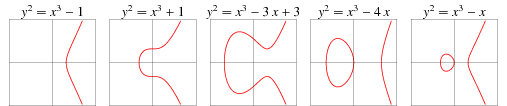Figure 1: 当 $$a,b$$ 取不同值时，椭圆曲线的形状变化（摘自：Wolfram MathWorld

### 1.1 椭圆曲线上的加法运算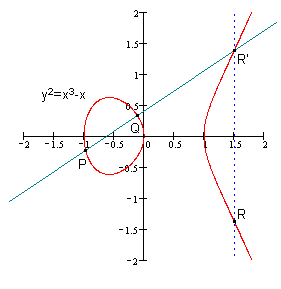Figure 2: 加法实例（不同点相加）： $$R=P+Q$$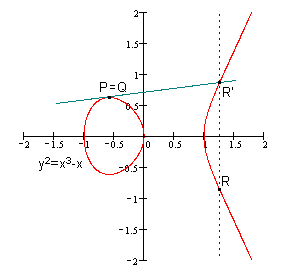Figure 3: 加法实例（相同点相加）： $$R=P+P=2P$$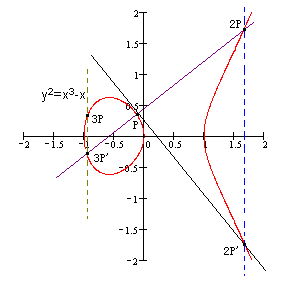Figure 4: $$P+P+P=2P+P=3P$$

### 1.2 加法运算公式

$y=m (x - x_Q) + y_Q$

$\begin{cases} y^2 = x^3 + ax + b \\ y=m (x - x_Q) + y_Q \\ \end{cases}$

$\begin{cases} x_R & = m^2 - x_P - x_Q \\ y_R & = - m (x_R - x_P) - y_P \\ \end{cases}$

$\begin{cases} x_R & = m^2 - x_P - x_Q \\ y_R & = - m (x_R - x_P) - y_P \\ \end{cases}$

#### 1.2.1 加法运算实例

\begin{aligned} m &= \frac{y_P - y_Q}{x_P - x_Q} = \frac{2 - 4}{1 - 3} = 1 \\ x_R &= m^2 - x_P - x_Q = 1^2 - 1 - 3 = -3\\ y_R &= - m (x_R - x_P) - y_P = - 1 (- 3 - 1) - 2 = 2 \\ \end{aligned}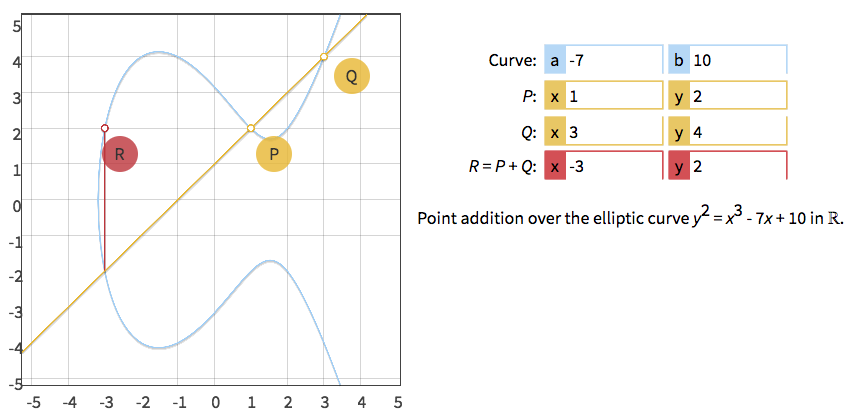Figure 5: $$R = P + Q$$

\begin{aligned} m &= \frac{3 x^2_P + a}{2y_P} = \frac{3 \cdot 1^2 - 7}{2 \cdot 2} = -1 \\ x_R &= m^2 - x_P - x_P = (-1)^2 - 1 - 1 = -1 \\ y_R &= - m (x_R - x_P) - y_P = 1 \cdot (-1 - 1) - 2 = -4 \\ \end{aligned}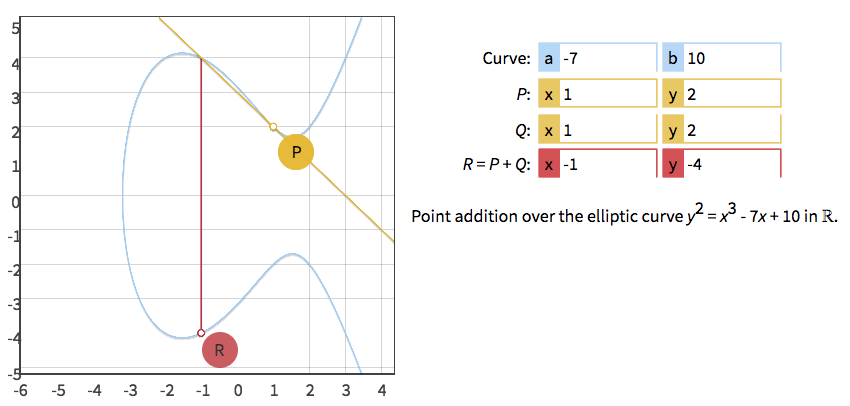Figure 6: $$R = 2P$$

## 2 密码学中的椭圆曲线

$\begin{array}{rcl} \left\{(x, y) \in \mathbb{R}^2 \right. & \left. | \right. & \left. y^2 = x^3 + ax + b, \right. \\ & & \left. 4a^3 + 27b^2 \ne 0\right\} \end{array}$

$\begin{array}{rcl} \left\{(x, y) \in (\mathbb{F}_p)^2 \right. & \left. | \right. & \left. y^2 \equiv x^3 + ax + b \pmod{p}, \right. \\ & & \left. 4a^3 + 27b^2 \not\equiv 0 \pmod{p}\right\} \cup\ \left\{ \mathcal{O} \right\} \end{array}$

Figure 7: Elliptic curve $$y^2 = x^3 − x$$ over finite field $$\mathbb{F}_{61}$$

7 中取两点 $$(4, 11), (4, 50)$$ ，验证如下：
\begin{aligned} x^3 − x = 4^3 - 4 = 60 \pmod {61} \\ y^2 = 11^2 = 121 \equiv 60 \pmod {61} \\ y^2 = 50^2 = 2500 \equiv 60 \pmod {61} \\ \end{aligned}

Figure 8: Elliptic curve $$y^2 = x^3 − x$$ over finite field $$\mathbb{F}_{71}$$

### 2.1 从椭圆曲线到“群”

(1) 封闭性： $$\forall a_1, a_2 \in A, \; a_1 + a_2 \in A$$
(2) 结合律： $$\forall a_1, a_2, a_3 \in A, \; (a_1 + a_2) + a_3 = a_1 + ( a_2 + a_3)$$
(3) 具有单位元： $$\exists e \in A, \; s.t. \; \forall a \in A, \; e + a = a + e = a$$
(4) 都有逆元素： $$\forall a \in A, \; \exists a^{-1} \in A, \; s.t. \; a + a^{-1} = e$$

\begin{aligned} x_R &= m^2 - x_P - x_Q \pmod p \\ y_R &= -m (x_R - x_P ) - y_P \pmod p \\ \end{aligned}

$P + \mathcal{O} = P$

$P + (-P) = \mathcal{O}$

$-P = (x_P, - y_P \bmod p)$

### 2.2 循环子群

$nP = \underbrace{P + P + \cdots + P}_{n \text{ times}}$

\begin{aligned} 0P &= \mathcal{O} \\ 1P &= (3, 6) \\ 2P &= (80, 10) \\ 3P &= (80, 87) \\ 4P &= (3, 91) \\ 5P &= \mathcal{O} \\ 6P &= (3, 6) \\ 7P &= (80, 10) \\ 8P &= (80, 87) \\ 8P &= (3, 91) \\ 10P &= \mathcal{O} \\ \cdots \\ \end{aligned}

## 3 Elliptic Curve Cryptography

### 3.1 Domain parameters

• The prime $$p$$ that specifies the size of the finite field.（注： $$p$$ 一般取很大的素数）
• The coefficients $$a$$ and $$b$$ of the elliptic curve equation.
• The base point $$G=(x_G, y_G)$$ that generates our subgroup.
• The order $$n$$ of the subgrouop.（注： $$n$$ 越大，越难暴力破解）
• The cofactor $$h=N/n$$ of the subgroup.（注： $$h$$ 一般为较小的正整数。它为 $$h=1$$ 时，循环子群就是椭圆曲线群本身）

#### 3.1.2 选择合适的椭圆曲线

• ANSI X9.62 (1999).
• IEEE P1363 (2000).
• SEC 2 (2000).
• NIST FIPS 186-2 (2000).
• ANSI X9.63 (2001).
• Brainpool (2005).
• NSA Suite B (2005).
• ANSSI FRP256V1 (2011).

### 3.2 Elliptic Curve Diffie-Hellman (ECDH)

Alice和Bob想进行通信，为了加密（基于效率考虑往往会采用对称密钥算法，如 AES 等）通信的数据，他们需要约定同一个密钥（称为“Shared Secret”）。

\begin{aligned} p &= \texttt{0xffffffff ffffffff ffffffff ffffffff ffffffff ffffffff fffffffe fffffc2f} \\ a &= \texttt{0} \\ b &= \texttt{7} \\ x_G &= \texttt{0x79be667e f9dcbbac 55a06295 ce870b07 029bfcdb 2dce28d9 59f2815b 16f81798} \\ y_G &= \texttt{0x483ada77 26a3c465 5da4fbfc 0e1108a8 fd17b448 a6855419 9c47d08f fb10d4b8} \\ n &= \texttt{0xffffffff ffffffff ffffffff fffffffe baaedce6 af48a03b bfd25e8c d0364141} \\ h &= \texttt{1} \\ \end{aligned}

1、从 $$\{1, 2, \cdots, n - 1\}$$ （ $$n$$ 在Domain parameters中指定的循环子群的阶）中随机选择一个数 $$d_A$$ 作为private key。
2、计算 $$Q_A=d_A G$$ （ $$G$$ 是Domain parameters中的Base Point）， $$Q_A$$ 就是public key。（注：这就是由私钥生成公钥的过程，直接按多次加法求解比较慢，可采用 Double-and-add 算法进行优化。这里有一个使用 Double-and-add 算法计算公钥的例子：https://bitcoin.stackexchange.com/questions/25024/how-do-you-get-a-bitcoin-public-key-from-a-private-key

### 3.3 公钥表达形式（uncompressed and compressed）

\begin{aligned} x_G &= \texttt{0x50863AD64A87AE8A2FE83C1AF1A8403CB53F53E486D8511DAD8A04887E5B2352} \\ y_G &= \texttt{0x2CD470243453A299FA9E77237716103ABC11A1DF38855ED6F2EE187E9C582BA6} \\ \end{aligned}

Q = 0450863AD64A87AE8A2FE83C1AF1A8403CB53F53E486D8511DAD8A04887E5B23522CD470243453A299FA9E77237716103ABC11A1DF38855ED6F2EE187E9C582BA6


### 3.4 Elliptic Curve Digital Signature Algorithm (ECDSA)

#### 3.4.1 ECDSA签名及验证过程

1. 对原始消息计算哈希（需要使用 Cryptographic hash function），得到 $$e=HASH(m)$$ ，把 $$e$$ 当做一个大整数。
2. 如果 $$e$$ 的比特长度大于 $$n$$ （ $$n$$ 在Domain parameters中指定的循环子群的阶）的比特长度，则把截断 $$e$$ ，只留下左边和 $$n$$ 同样比特长的部分，记为 $$z$$ ，后面把它当做大整数进行运算。

Alice签名过程：

1. 从 $$\{1, 2, \cdots, n - 1\}$$ 中随机选择一个数，记为 $$k$$ ；
2. 计算 $$P = k G$$ （其中 $$G$$ 是Domain parameters）；
3. 计算整数 $$r = x_P \pmod n$$ （其中 $$x_P$$ 是上一步计算的点 $$P$$ 的横坐标）；
4. 如果 $$r=0$$ ，则随机选择另外一个 $$k$$ ，重新计算前面两步；
5. 计算整数 $$s= k^{-1}(z + rd_A) \pmod n$$ （其中 $$d_A$$ 是Alice的私钥， $$k^{-1}$$ 是满足 $$k k^{-1} = 1 \pmod n$$ 的整数，整数 $$z$$ 是摘要消息）；
6. 如果 $$s=0$$ ，则随机选择另外一个 $$k$$ ，重新计算前面步骤。

Bob验证签名的过程：

1. 计算整数 $$u_1 = s^{-1} z \pmod n$$ （其中 $$s^{-1}$$ 是满足 $$s s^{-1} = 1 \pmod n$$ 的整数）；
2. 计算整数 $$u_2 = s^{-1} r \pmod n$$ ；
3. 计算点 $$P = u_1 G + u_2 Q_A$$ （其中 $$Q_A$$ 是Alice的公钥）。
4. 如果 $$r = x_P \pmod n$$ （这里 $$x_P$$ 是上一步计算的点 $$P$$ 的横坐标）成立，则验证签名通过，否则验证失败。
##### 3.4.1.1 ECDSA正确性证明

\begin{aligned} P &= u_1 G + u_2 Q_A \\ & = u_1 G + u_2 d_A G \\ & = (u_1 + u_2 d_A) G \\ & = (s^{-1} z + s^{-1} r d_A) G \\ & = s^{-1} (z + r d_A) G \\ \end{aligned}

\begin{aligned} P & = k G \\ & = s^{-1} (z + r d_A) G \\ \end{aligned}

#### 3.4.2 ECDSA的Python实现

#!/usr/bin/env python3
# -*- coding: utf-8 -*-
# 代码摘自：https://github.com/andreacorbellini/ecc/blob/master/scripts/ecdsa.py

import collections
import hashlib
import random

EllipticCurve = collections.namedtuple('EllipticCurve', 'name p a b g n h')

curve = EllipticCurve(
'secp256k1',
# Field characteristic.
p=0xfffffffffffffffffffffffffffffffffffffffffffffffffffffffefffffc2f,
# Curve coefficients.
a=0,
b=7,
# Base point.
g=(0x79be667ef9dcbbac55a06295ce870b07029bfcdb2dce28d959f2815b16f81798,
# Subgroup order.
n=0xfffffffffffffffffffffffffffffffebaaedce6af48a03bbfd25e8cd0364141,
# Subgroup cofactor.
h=1,
)

# Modular arithmetic ##########################################################

def inverse_mod(k, p):
"""Returns the inverse of k modulo p.
This function returns the only integer x such that (x * k) % p == 1.
k must be non-zero and p must be a prime.
"""
if k == 0:
raise ZeroDivisionError('division by zero')

if k < 0:
# k ** -1 = p - (-k) ** -1  (mod p)
return p - inverse_mod(-k, p)

# Extended Euclidean algorithm.
s, old_s = 0, 1
t, old_t = 1, 0
r, old_r = p, k

while r != 0:
quotient = old_r // r
old_r, r = r, old_r - quotient * r
old_s, s = s, old_s - quotient * s
old_t, t = t, old_t - quotient * t

gcd, x, y = old_r, old_s, old_t

assert gcd == 1
assert (k * x) % p == 1

return x % p

# Functions that work on curve points #########################################

def is_on_curve(point):
"""Returns True if the given point lies on the elliptic curve."""
if point is None:
# None represents the point at infinity.
return True

x, y = point

return (y * y - x * x * x - curve.a * x - curve.b) % curve.p == 0

def point_neg(point):
"""Returns -point."""
assert is_on_curve(point)

if point is None:
# -0 = 0
return None

x, y = point
result = (x, -y % curve.p)

assert is_on_curve(result)

return result

"""Returns the result of point1 + point2 according to the group law."""
assert is_on_curve(point1)
assert is_on_curve(point2)

if point1 is None:
# 0 + point2 = point2
return point2
if point2 is None:
# point1 + 0 = point1
return point1

x1, y1 = point1
x2, y2 = point2

if x1 == x2 and y1 != y2:
# point1 + (-point1) = 0
return None

if x1 == x2:
# This is the case point1 == point2.
m = (3 * x1 * x1 + curve.a) * inverse_mod(2 * y1, curve.p)
else:
# This is the case point1 != point2.
m = (y1 - y2) * inverse_mod(x1 - x2, curve.p)

x3 = m * m - x1 - x2
y3 = y1 + m * (x3 - x1)
result = (x3 % curve.p,
-y3 % curve.p)

assert is_on_curve(result)

return result

def scalar_mult(k, point):
"""Returns k * point computed using the double and point_add algorithm."""
assert is_on_curve(point)

if k % curve.n == 0 or point is None:
return None

if k < 0:
# k * point = -k * (-point)
return scalar_mult(-k, point_neg(point))

result = None

while k:
if k & 1:

# Double.

k >>= 1

assert is_on_curve(result)

return result

# Keypair generation and ECDSA ################################################

def make_keypair():
"""Generates a random private-public key pair."""
private_key = random.randrange(1, curve.n)
public_key = scalar_mult(private_key, curve.g)

return private_key, public_key

def hash_message(message):
"""Returns the truncated SHA521 hash of the message."""
message_hash = hashlib.sha512(message).digest()
e = int.from_bytes(message_hash, 'big')

# FIPS 180 says that when a hash needs to be truncated, the rightmost bits
# should be discarded.
z = e >> (e.bit_length() - curve.n.bit_length())

assert z.bit_length() <= curve.n.bit_length()

return z

def sign_message(private_key, message):
z = hash_message(message)

r = 0
s = 0

while not r or not s:
k = random.randrange(1, curve.n)
x, y = scalar_mult(k, curve.g)

r = x % curve.n
s = ((z + r * private_key) * inverse_mod(k, curve.n)) % curve.n

return (r, s)

def verify_signature(public_key, message, signature):
z = hash_message(message)

r, s = signature

w = inverse_mod(s, curve.n)
u1 = (z * w) % curve.n
u2 = (r * w) % curve.n

x, y = point_add(scalar_mult(u1, curve.g),
scalar_mult(u2, public_key))

if (r % curve.n) == (x % curve.n):
return 'signature matches'
else:
return 'invalid signature'

print('Curve:', curve.name)

private, public = make_keypair()
print("Private key:", hex(private))
print("Public key: (0x{:x}, 0x{:x})".format(*public))

msg = b'Hello!'
signature = sign_message(private, msg)

print()
print('Message:', msg)
print('Signature: (0x{:x}, 0x{:x})'.format(*signature))
print('Verification:', verify_signature(public, msg, signature)) # 验证通过

msg = b'Hi there!'                # 使用不同的消息，会验证失败
print()
print('Message:', msg)
print('Verification:', verify_signature(public, msg, signature))

private, public = make_keypair()  # 使用不同的公钥，会验证失败

msg = b'Hello!'
print()
print('Message:', msg)
print("Public key: (0x{:x}, 0x{:x})".format(*public))
print('Verification:', verify_signature(public, msg, signature))


### 3.5 Elliptic Curve Integrated Encryption Scheme (ECIES)

ECIES is a hybrid encryption system proposed by Victor Shoup in 2001. ECIES has been standardized in ANSI X9.63, IEEE 1363a, ISO/IEC 18033-2, and SECG SEC-1.

9 是四种不同ECIES方案的比较（摘自 A Comparison of the Standardized Versions of ECIES ）。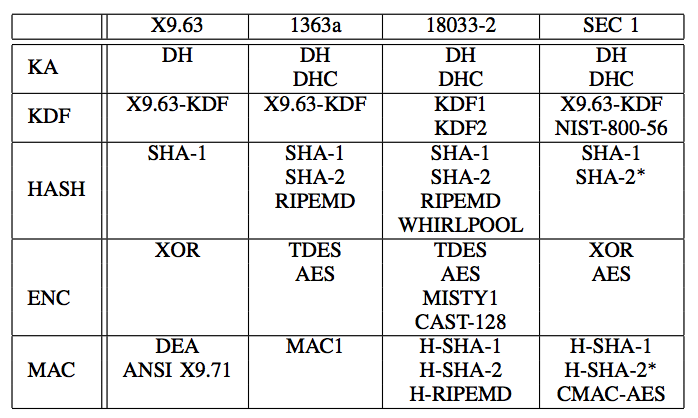Figure 9: 四种ECIES方案

Created: <2018-05-01 Tue 00:00>

Last updated: <2018-09-12 Wed 17:31>

Creator: Emacs 25.3.1 (Org mode 9.1.4)